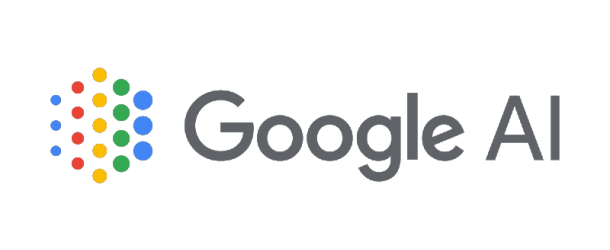(QuantaMagazine) Quantum computers theoretically can do anything that a classical computer can. In practice, however, the quantumness in a quantum computer makes it nearly impossible to efficiently run some of the most important classical algorithms.
In April, a software engineer at Google AI Quantum in Santa Barbara, California, described a quantum version of a classical algorithm for quickly multiplying very large numbers. Classical computers have been running this algorithm for a long time. Before Gidney’s paper it was unclear whether it would be possible to retrofit it for quantum machines.
The multiplication algorithm is part of a class of nearly ubiquitous algorithms in computer science. Gidney expects that his new technique will allow quantum computers to implement this class of algorithms, which until now appeared to be too cumbersome to be used in a quantum machine.
Gridley used the Karatsuba method developed in 1960 by a Russian mathematician Anatoly Karatsuba. His method involves splitting long numbers into shorter numbers. To multiply two eight-digit numbers, for example, you would first split each into two four-digit numbers, then split each of these into two-digit numbers. You then do some operations on all the two-digit numbers and reconstitute the results into a final product. For multiplication involving large numbers, the Karatsuba method takes far fewer steps than the grade-school method.
Gidney described a quantum way of implementing Karatsuba multiplication that doesn’t impose huge memory costs. Gidney expects that his method will work for adapting many classical recursive algorithms to quantum computers.

• #### Latest Blog Posts